/

### The F distribution

The F distribution is also known as Snedecor’s F or the Fisher-Snedecor distribution. It commonly arises in statistical tests linked to analysis of variance.

 Distribution name F distribution Common notation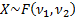Parameters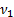= degrees of freedom (first) (positive integer)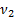= degrees of freedom (second) (positive integer) Domain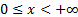Probability density function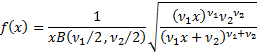Cumulative distribution function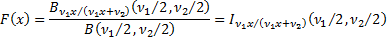Mean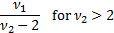Variance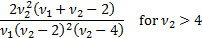Skewness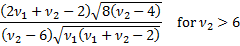(Excess) kurtosis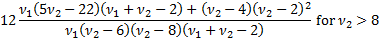Characteristic function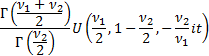Where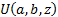is the confluent hypergeometric function of the second kind Other comments The F distribution is a special case of the Pearson type 6 distribution. It is also a particular example of the beta prime distribution.   If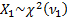and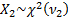are independent random variables then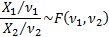Its mode is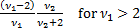. There is no simple closed form for the median.

Nematrian web functions

Functions relating to the above distribution may be accessed via the Nematrian web function library by using a DistributionName of “f”. Functions relating to a generalised version of this distribution including additional location (i.e. shift) and scale parameters may be accessed by using a DistributionName of “f4”, see also including additional shift and scale parameters. For details of other supported probability distributions see here.